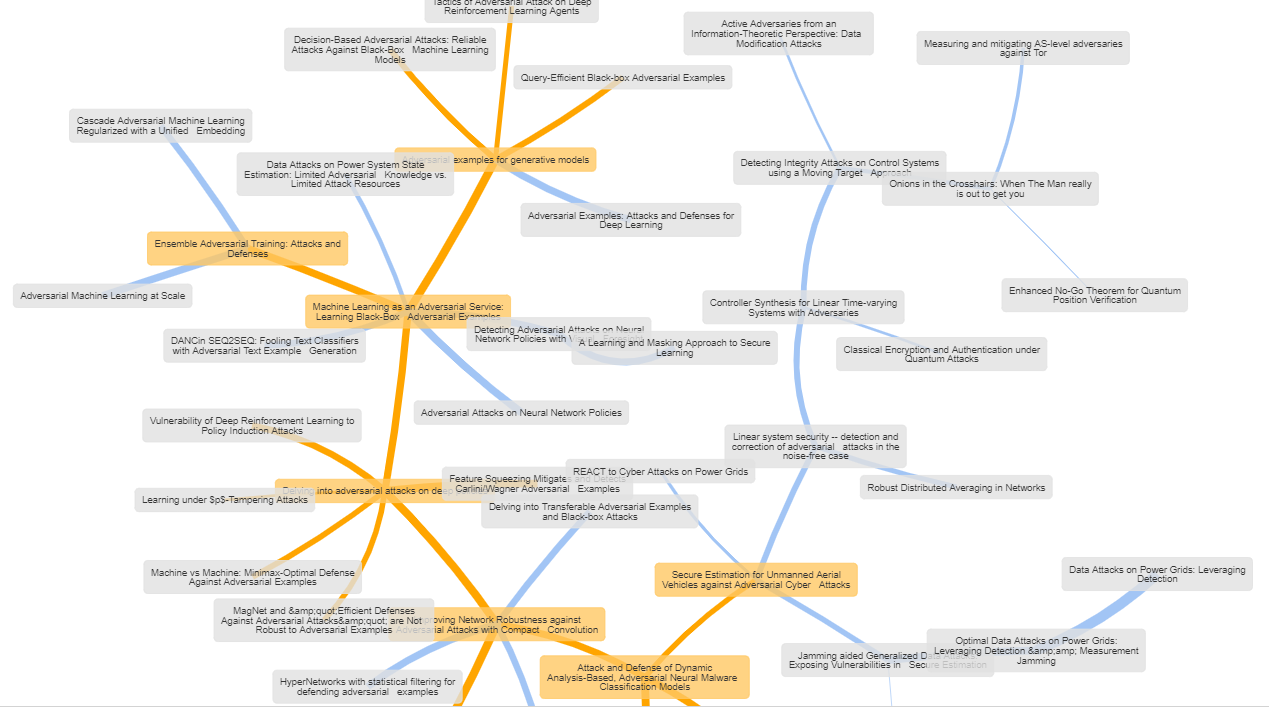# Interactive Search 1

22 Dec 2017

Problem: I don't want to go through the 100 returned results but want to be fairly confident that say, the 10 results I looked at are close to best matches.

Given some matrix,D, where each row is a weights matrix of some factored document's representation (e.g. by local dirichlet allocation, non-negative matrix factorization, reflective random indexing—Of those, I've found RRI to be best for my purposes; searching through 50-100 pages). And where each row has L2-Norm=1.

let $$\mathbf G=\mathbf D \mathbf D^{\mathrm {T}}$$

G is a graph (and also a Gramian matrix).

$$\mathbf T = \mathrm{MaxSpanningTree}(\mathbf G)$$

T helps us efficiently update a sampling strategy. Nodes are connected by similarity. For step 1, we sample a random node (which are all pages, and optionally can sample a paragraph from a page). Human feedback is: neutral, strong positive, strong negative, eliminate, positive,negative). Sampled neighbor gets reward as well as neighbor's neighbors (attenuated by degree and normalized relatedness).This information propagates through the local section of the graph which in turn rebalances the sampling probability of which page will be sampled next.

If sampling a paragraph at a time, first sample page. Each page has a similar tree calculated comparing its paragraphs. Look up page's tree and sampler to sample a paragraph. On reward, propagate through page's tree. Then attenuated reward through page sampler.

Sampling and update done with probability monad simulating min-regret learning.

More soon.

The image is a tree calculated by searching arxiv for 50 results on adversarial attack, and using RRI on the abstracts. The graph is useful in and of itself as an artifact. At a glance, I can tell the topics are: deep learning, power grids, quantum communication and Tor. I would 100% get sea-sick looking through 50 results to ascertain this. Works on any language (for chinese, the dumbest thing of changing split to words => split to characters is still good), and calculated from scratch on results.

At a finer level, the tree paths seem to follow the particular approach of the deep learning papers. The example is done on just the abstract of 50 papers and so has a few odd placements. It does even better on at least page length inputs. The method lives and dies by the original matrix construction.

###Sketch

 v = Neigbours(lookup[d])

w = List.map Neigbours v / v

sample page ~ Pages
sample p ~ uniform 0 1
use v and w to propogate reward